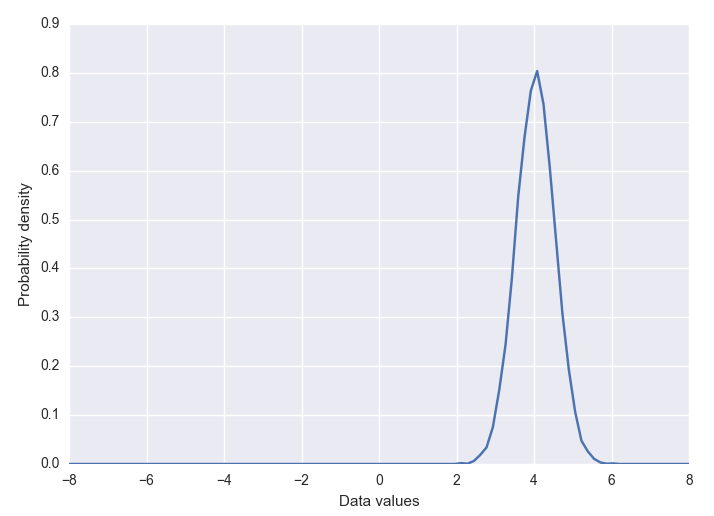# GAN with an example

For a deeper understanding of how GANs work, we'll use a GAN to solve a simple problem in TensorFlow, namely, learning to approximate a one-dimensional Gaussian distribution.

First, we will create the actual data distribution, a simple Gaussian with a mean of four and standard deviation of 0.5. It has a sample function that returns a given number of samples (sorted by value) from the distribution. The data distribution that we learn will look like the following diagram:Generator input noise distribution is also defined with a similar sample function used for actual data.

Both generator and discriminator networks are very ...

Get Neural Network Programming with TensorFlow now with the O’Reilly learning platform.

O’Reilly members experience books, live events, courses curated by job role, and more from O’Reilly and nearly 200 top publishers.